• 引言 算法在工程中的应用越来越广泛，...为了体验tensorflow的开发模式，笔者使用tensorflow实现了一个基于LSTM算法的预测实例，与大家分享。 LSTM的前世今生 在LSTM之前，我们先介绍另一个如雷贯耳的名词RNN(...


引言
算法在工程中的应用越来越广泛，tensorflow在工业界大放异彩。笔者的项目也使用了LSTM算法的预测能力，大大降低了运用的成本，提高了运营效率。
为了体验tensorflow的开发模式，笔者使用tensorflow实现了一个基于LSTM算法的预测实例，与大家分享。
LSTM的前世今生
在LSTM之前，我们先介绍另一个如雷贯耳的名词RNN(Recurrent Neural Networks)，中文名是递归神经网络。LSTM是对RNN隐含层的改进。RNN最主要的用途是处理序列数据，且该序列的当前输出与前面的输出也有关。比如，要分析一段文本的含义时，当前词汇与上一个词汇是有关系的，并不是孤立存在的，上文对当前的输出产生了影响。因此RNN在NLP（Natural Language Processing）中获得非常成功的应用。
LSTM因为《Long short-term memory》这篇论文为世人所熟知，LSTM的出现是为了解决RNN无法有效处理长期记忆（Long Term Dependencies）的问题。
标准RNN在进行长期记忆计算会产生‘梯度消失或者梯度膨胀问题’（原作者描述为“vanishing or exploding gradients”），即RNN的梯度数据会在若干次递归计算后，由于反向多次求导的递归过程中产生的计算误差的叠加，导致梯度消失或者梯度膨胀。粗略的说，‘梯度消失’的表现为很多个小于1的项连乘就很快的逼近零。
LSTM是如何做到避免梯度消失，简单的说，LSTM在神经元的状态转移时，不对状态值添加scale参数或矩阵变换。同时，引入‘门函数’，如输入门，输出门，遗忘门，控制输入信息、状态转移，以及输出信息的有选择性地表达。
tensorflow
tensorflow是google发布的一个使用数据流图进行数值计算的开源软件库，计算的对象称为张量，即多维数组。已经发布了可用于生产环境的1.0版本, 被工业界广泛用于机器学习和深度学习的研究和算法应用开发。
官方主页：https://www.tensorflow.org/

预测场景
LSTM非常适用于基于时序数据的预测，比如预测未来的股票行情，预测未来某个时间段的交通情况，预测未来的房价等。
本文使用房价数据作为训练样本，因为人在对房价进行预测时，基本上都会参考近期房价和历史趋势，对未来的价格作出一个预判。也就是说，历史上的房价数据，是会对未来的房价产生重大影响的，即当前输出受历史输出的影响，则房价的预测符合LSTM的应用优势。
定义LSTM模型
本文使用单层的LSTM模型对数据进行预测，更为复杂的多层LSTM模型也是基于单层LSTM模型进行扩展。定义一个基本的LSTM模型，需要定义如下几个部分：    1. 定义时间步, 定义每个输入数据与前多少个有序的输入的数据有关联
2. 定义隐层神经元的数量
3. 定义每批训练样本数
4. 定义输入层维度、权重、偏置
5. 定义输出层维度、权重、偏置
6. 定义学习率，学习率越小，越容易陷入局部最优结果，学习率越大，相邻两次训练结果间的抖动越大
7. 定义损失函数
8. 定义训练次数
9. 构建训练数据集
10. 构建测试数据集
获取数据集
在预测房价之前，需要先获取房价的数据，本文的数据来源为某房地产中介平台的交易数据。
如何使用python编写一个爬虫精确爬取数据，详见另一篇文章：Python爬虫实战, 这也是为什么使用房价作为实例场景的原因，因为这个数据已经被爬取过了。
基于Tensorflow的LSTM实现
基于LSTM的demo代码实现在github上发布，传送门：https://github.com/huntertan/lstm-spider/tree/master/prediction

关键文件

PricePredictor.py
LSTM预测模型实现

chargeInfo.txt
房价数据

算法效果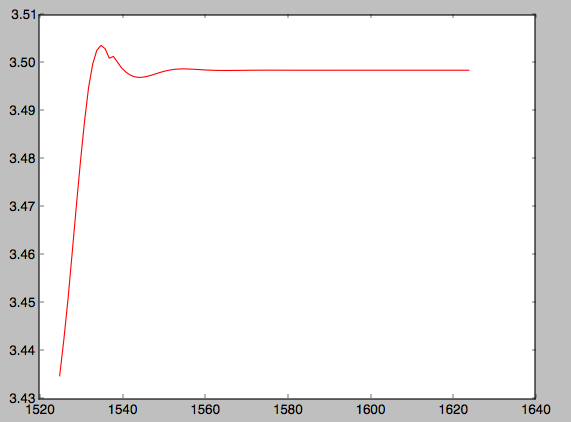纵坐标数值的单位为 万RMB结束语
本文实现的demo离实际的工程应用还有差距，仅供体验和参考
算法的应用在某些场景中能够带来令人惊喜的效果，但在一个新的场景或者应用领域中，算法的效果具有很大的不确定性。
算法的基础理论晦涩难懂，二次开发和准确应用的门栏都相对较高，在工程应用过程中，不免会碰到算法持续改进了几个月，却在复杂的业务场景中表现不佳，无法真正上线应用的情况。
由于算法的不确定性，在进行严格排期的工程实施中，要准确回答‘使用算法解决xxx问题，需要几个人日’的问题，的确是个颇为困难的事。
希望越来越多成功的算法应用，能够不断改善人们的体验，解放人们的想象力，创造更加美好的生活。

展开全文• 1.什么是ECS 弹性云服务器（Elastic Cloud Server，ECS）是由CPU、内存、操作系统、云硬盘组成的最基础的计算组件...分析过往租户对ECS实例请求的行为的规律，针对性地使用预测算法，为云平台的提供一个精准的需虚拟...
1.什么是ECS
弹性云服务器（Elastic Cloud Server，ECS）是由CPU、内存、操作系统、云硬盘组成的最基础的计算组件。开通只需要指定CPU、内存、操作系统、规格、登录鉴权方式即可，同时也可以根据需求随时调整弹性云服务器规格，为您打造一个高效、可靠、安全的计算环境。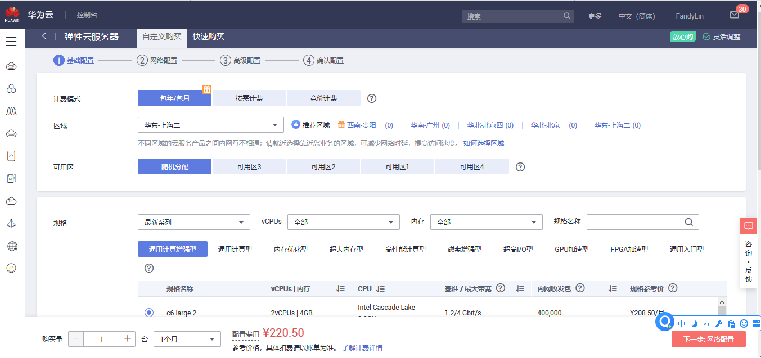2、虚拟机预测
分析过往租户对ECS实例请求的行为的规律，针对性地使用预测算法，为云平台的提供一个精准的需虚拟机实例预测服务，将虚拟机预测实例结果交付，云平台会根据虚拟机预测结果以最小成本选择物理主机将所有虚拟机安排放置。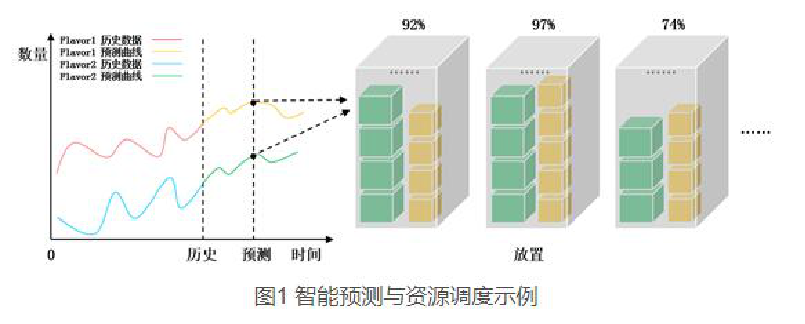2.1 虚拟机预测——预测内容的要求(input.txt)
第一行：物理主机参数
CPU核数 内存大小（GB） 硬盘大小（GB）
第三行：需要预测的虚拟机类型数目
第四行开始：需要预测的虚拟机类型参数
虚拟机名称 CPU核数 内存大小（MB）
倒数两行：预测的时间范围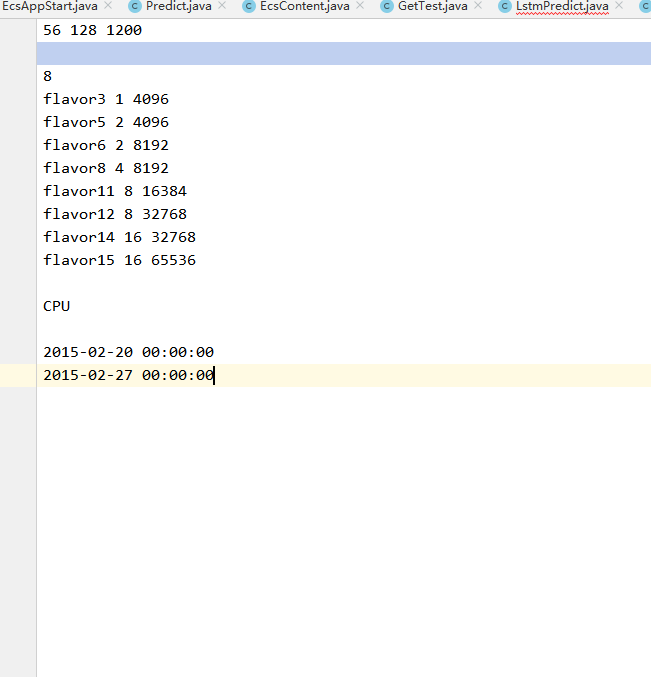2.2 虚拟机预测——过往虚拟机实例数据集（data.txt）
第一列：虚拟机id
第二列：虚拟机型号
第三列：虚拟机部署的时间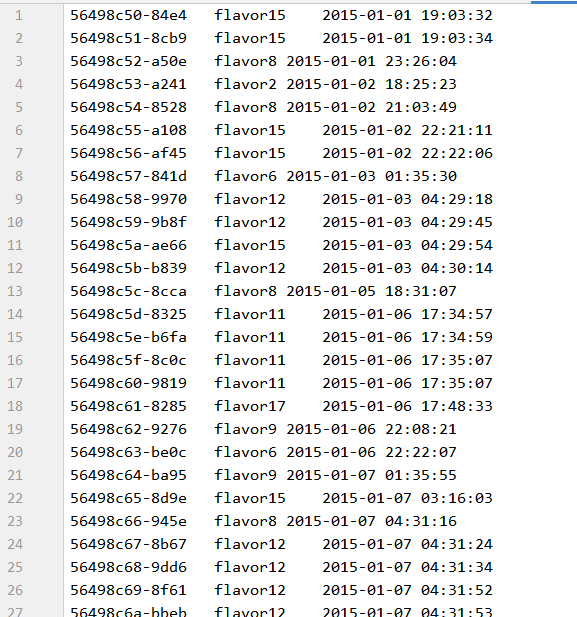2.3 虚拟机预测——数据的表示（预处理）
将data.txt中的过往虚拟机实例，结合input.txt中需要预测的虚拟机类型要求
构建矩阵：行代表天，列代表该天每种虚拟机类型的实例个数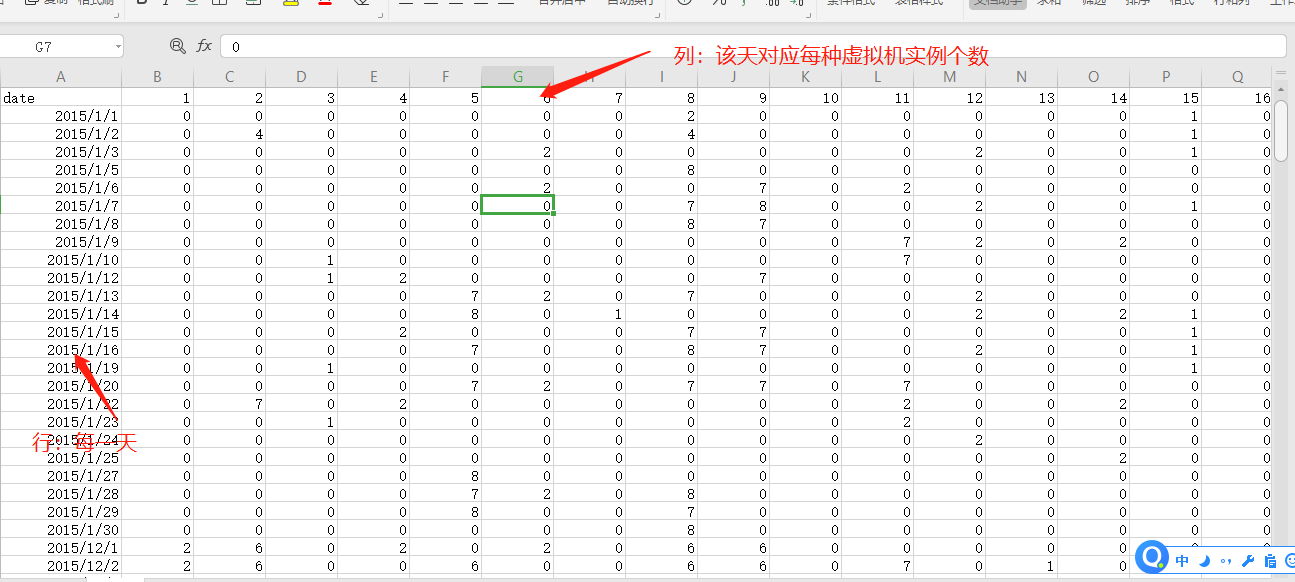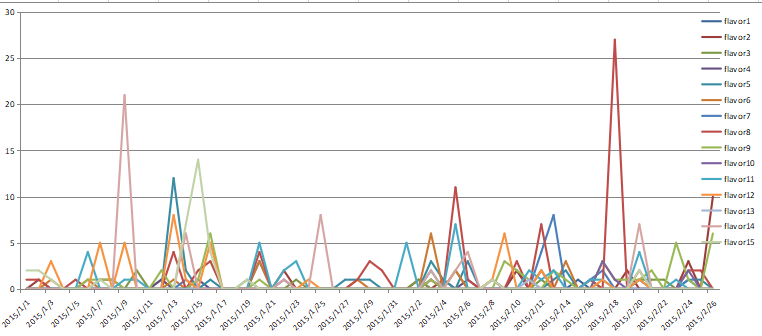2.4 虚拟机预测——预测精度计算规则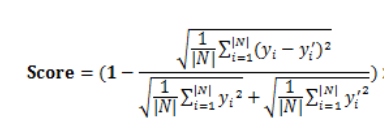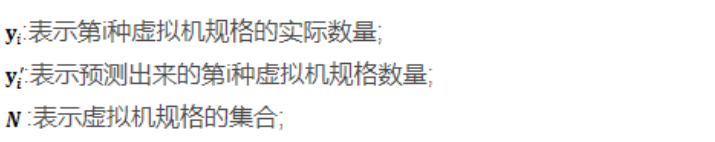3、使用lstm的核心思路
1、根据前n—>m天的时间序列每种虚拟机实例情况预测n+1—>m+1天虚拟机实例情况
2、数据降噪处理：箱形图最大值点替代异常值
程序流程图：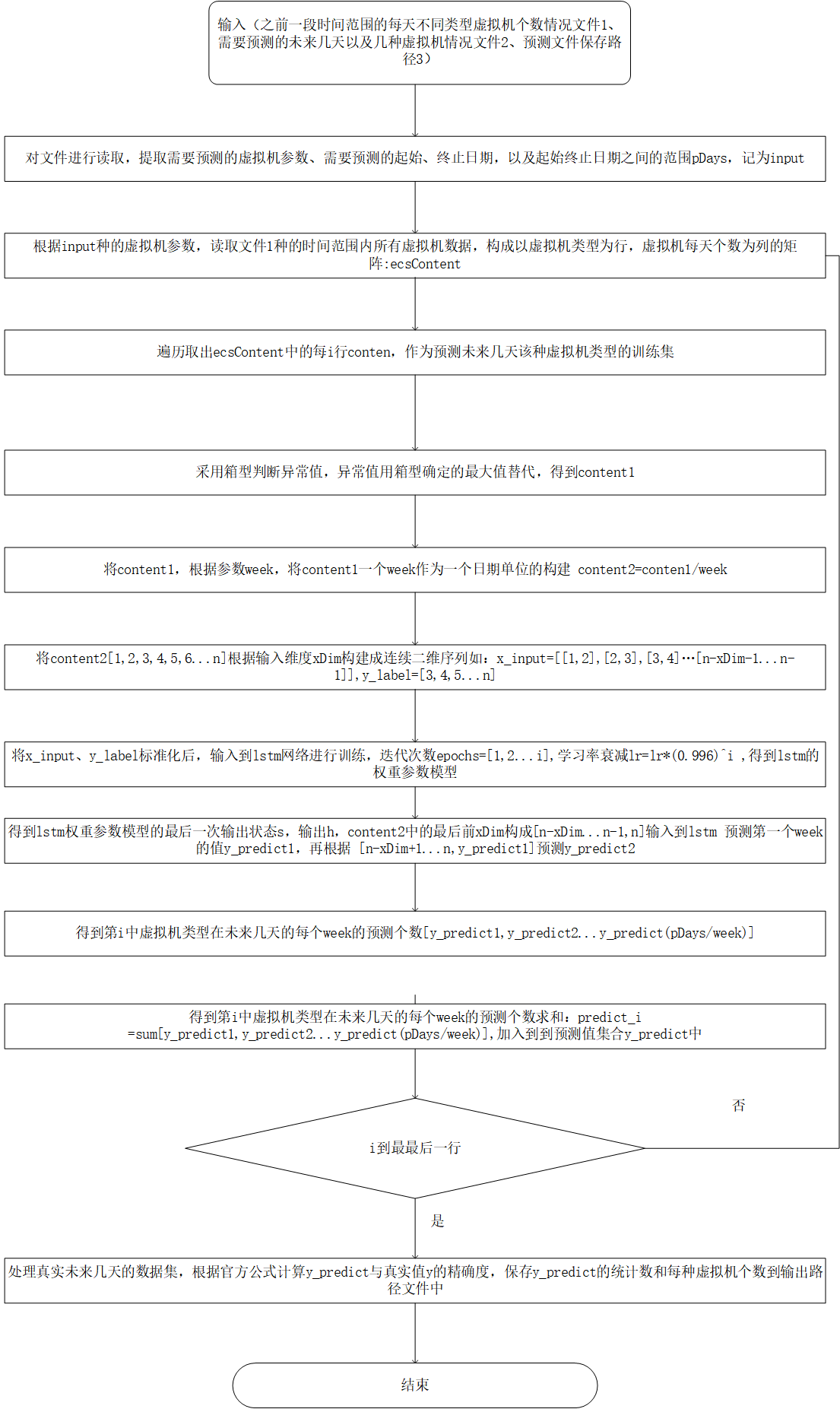展开全文自然语言处理 神经网络 深度学习 tensorflow
• 　感觉很多chatbot的博文都是直接拿seq2seq开刀，上来就堆了一堆RNN（或者LSTM，Attention）模型的原理和公式。本篇从初学者的角度出发更想将机器学习基础（目标函数，优化方法，正则化等思想）贯穿始终。并结合...
一、总体分析

感觉很多chatbot的博文都是直接拿seq2seq开刀，上来就堆了一堆RNN（或者LSTM，Attention）模型的原理和公式。本篇从初学者的角度出发更想将机器学习基础（目标函数，优化方法，正则化等思想）贯穿始终。并结合Tensorboard可视化tensorflow中相关的模型算法。
在Machine Learning by Mitchell(1997)中，给出了机器学习的一个简洁定义：“对于某类任务 T$T$$T$ 和性能度量 P$P$$P$，一个计算机程序被认为可以从经验 E$E$$E$ 中学习是指，通过经验 E$E$$E$ 改进后，它在任务 T$T$$T$ 上由性能度量 P$P$$P$ 衡量的性能有所提升 ”。所以对于我们的非任务导向型的对话系统（chatbot）而言，也可以从这三维度展开讨论。

1.1 任务 T$T$$T$

机器学习任务定义为机器学习系统应该如何处理样本。样本是指我们从某些希望机器学习系统处理的对象或事件中收集到的已经量化的特征的集合。在上一篇博文中预处理成问答对并用word2vec模型训练后的词向量即是我们chatbot系统的样本数据。机器学习的学习任务有很多种，如分类，回归，转录，机器翻译，异常值检测，密度估计（学习样本采样空间的概率密度函数）等。我们这里的任务大概可以描述为给指定问句生成对答语句。

1.2 性能度量 P$P$$P$

对于诸如分类，转录之类的任务，通常度量模型的准确率（即模型输出正确结果的样本比例）。回归之类的任务通常用模型输出和样本实际目标值 y$y$$y$ 之间的均方误差 MSE$MSE$$MSE$ 来衡量。对于密度估计类任务，更通常的是评估模型生成的概率密度函数（模型分布pmodel${p}_{model}$$p_{model}$）和样本实际概率密度函数（经验分布p̂data${\stackrel{^}{p}}_{data}$$\hat p_{data}$）之间的相似性，通常用KL散度来衡量。
DKL(p̂data||pmodel)=Ex∼p̂data[logp̂data(x)−logpmodel(x)]${D}_{KL}\left({\stackrel{^}{p}}_{data}||{p}_{model}\right)={E}_{x\sim {\stackrel{^}{p}}_{data}}\left[\mathrm{log}{\stackrel{^}{p}}_{data}\left(x\right)-\mathrm{log}{p}_{model}\left(x\right)\right]$$D_{KL}(\hat p_{data} || p_{model}) = E_{x\sim \hat p_{data}}[\log \hat p_{data}(x)-\log p_{model}(x)]$
其中logp̂data(x)$\mathrm{log}{\stackrel{^}{p}}_{data}\left(x\right)$$\log \hat p_{data}(x)$仅涉及数据生成过程，和模型无关。这意味着最小化KL散度时，只需要最大化 Ex∼p̂data[logpmodel(x)]${E}_{x\sim {\stackrel{^}{p}}_{data}}\left[\mathrm{log}{p}_{model}\left(x\right)\right]$$E_{x\sim \hat p_{data}}[\log p_{model}(x)]$ ，这也即是最大似然估计。

1.３ 经验 E$E$$E$

根据学习过程中的不同经验，机器学习算法大致分为无监督学习算法和监督学习算法。通常的无监督学习任务除了聚类之外还有一些需要学习生成数据集的整个概率分布，显示地比如密度估计，或是隐式地比如合成或去噪等。监督学习算法训练含有很多特征的数据集，不过数据集中的样本都有一个目标值。就我们当前的任务和数据集来说，显然是监督学习过程，每个问答对(question-answer)中的answer部分就是模型要匹配的目标值。

总结以上三点，对我们要构建的机器学习系统就是针对为给指定问句生成对答语句这个任务，使用监督学习算法构建模型，针对每个question用模型的输出去拟合实际的answer。并可以使用最小化均方误差作为性能度量标准来不断优化模型参数。

二、模型算法

第一小节从机器学习整体过程入手给出了Chatbot的大致框架，接下来就需要针对任务的具体特性来分析。根据question生成answer总体上来说是一个Seq2Seq模型（也称为Encoder-Decoder模型），在上一篇博文中对question和answer经过中文分词并生成词向量之后，其实question和answer就分别是问题词向量序列和回答词向量序列了。这种结构最重要的地方在于输入序列和输出序列的长度是可变的（参考The Unreasonable Effectiveness of Recurrent Neural Networks）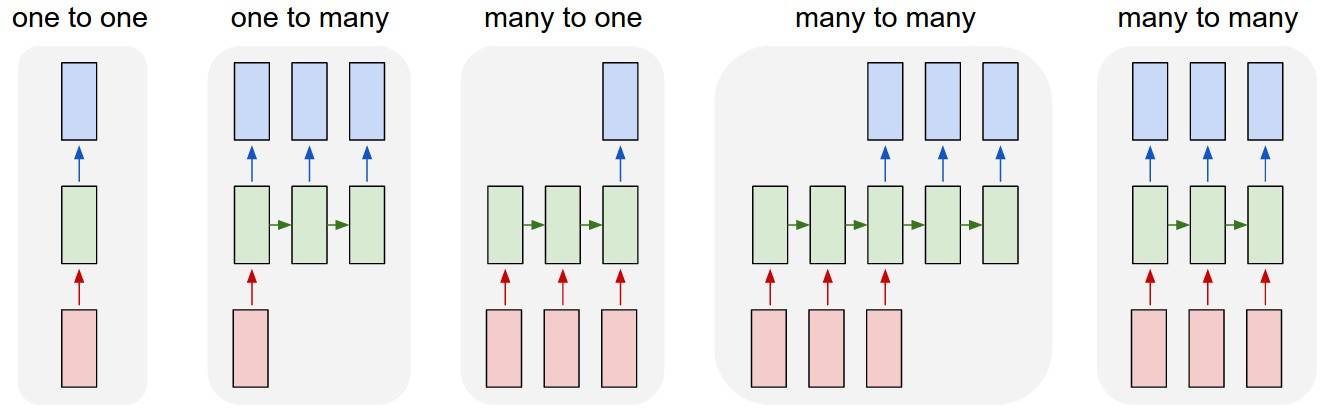Fig 2-1 Encoder-Decoder模型的多种输入输出形式
论文Sequence to Sequence Learning with Neural Networks中给出了直观的Seq2Seq模型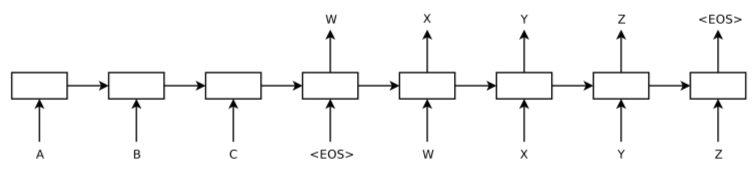Fig 2-2 经典Seq2Seq模型
其中ABC是输入语句，WXYZ是输出语句，EOS是标识一句话结束，图中的训练单元是lstm，其核心在于 cell 和 结构图上面的那条横穿的水平线。cell 状态的传输就像一条传送带，向量从整个 cell 中穿过，只是做了少量的线性操作。这种结构能够很轻松地实现信息从整个 cell 中穿过而不做改变。从而可以保持长时期记忆。更多基础原理和算法设计可参考经典文献Understanding LSTM Networks。，所以能够根据输入的多个字来确定后面的多个字。显示融合了LSTM模型的并区分出Encoder和Decoder的示意图如下：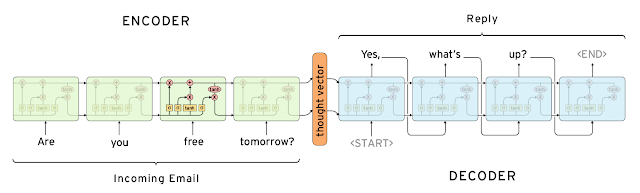Fig 2-3 融合LSTM的seq2seq模型
图中的 Encoder 和 Decoder 都只展示了一层的普通的 LSTMCell。从上面的结构中，我们可以看到，整个模型结构还是非常简单的。 EncoderCell 最后一个时刻的状态[cXT,hT]$\left[{c}_{{X}_{T}},{h}_{T}\right]$$[c_{X_T}, h_T]$就是整个句子的语义向量，它将作为 DecoderCell 的初始状态。然后在 DecoderCell 中，每个时刻的输出将会作为下一个时刻的输入。以此类推，直到 DecoderCell 某个时刻预测输出特殊符号<END>$$$$结束。

三、基于Tensorflow的Chatbot实例

tensorflow自带的seq2seq模型基于one-hot的词嵌入向量（把每个单词按顺序编号，每个词就是一个很长的向量，向量的长度等于词表的大小，只有对应位置上的数字编号为1，其余位置为0.在实际应用中一般采用稀疏矩阵的表示方式）。这种表示方法不足以表示词与词之间的关系（因为任何两个词向量之间的L2$L2$$L2$距离都是2‾√$\sqrt{2}$$\sqrt 2$，体现不出相似词）。因此我们之前预处理是采用基于word2vec的多维词向量。这里给出一个基于one-hot的词嵌入向量的英文对话系统的开源项目供参考，后面都将围绕word2vec多维词向量展开。
我们是使用的看了很多经典论文中阐释的Seq2Seq模型，Decoder中每个LSTMCell的输入都是上一个LSTMCell的输出（第一个除外，Decoder中第一个LSTM的输入是特殊的起始向量 如Start$Start$$Start$）。但是在实际训练中，Decoder输出是一个词一个词依次输出的，我们没法保证上一个Decoder LSTMCell的输出就是实际answer中的正确输出，因而这样的输出提供给下一个Decoder LSTMCell的输入，只会让误差越来越大，或者说算法需要更长的训练时间。因此我们直接用answer中 T$T$$T$ 时刻的实际值yt${y}_{t}$$y_{t}$作为第T+1$T+1$$T+1$个Decoder LSTMCell的输入。也即对于 Fig 2-2  所示的question=”ABC”，answer=”WXYZ”的情况下。我们模型实际输入为InputX$InputX$$InputX$=”ABCSWXY”（其中InputXEncoder${InputX}_{Encoder}$${InputX}_{Encoder}$=”ABC”, InputXDecoder${InputX}_{Decoder}$${InputX}_{Decoder}$=”SWXY”,S表示Decoder的起始输入Start），模型要拟合的输出为TargetY$TargetY$$TargetY$={WXYZ}。参考文献【6】里给了一张较为清晰的全流程手绘图，如下：Fig 3-1 全流程手绘图
基于Tensorflow的Chatbot实例核心代码如下：

class Seq2Seq(object):
def __init__(self, word_vector_model_path, seq2seq_model_path, input_file, word_vec_dim=200, max_seq_len=16):
"""
:param word_vector_model_path: 已经训练好的word2vec词向量模型路径
:param seq2seq_model_path: seq2seq模型路径
:param input_file: 已经处理好的问答对源文件
:param word_vec_dim: 词向量长度
:param max_seq_len: 最大序列长度
"""
self.word_vector_model_path = word_vector_model_path
self.input_file = input_file
self.word_vec_dim = word_vec_dim
self.max_seq_len = max_seq_len
self.seq2seq_model_path = seq2seq_model_path
self.question_seqs = []  # 问题序列集
self.answer_seqs = []  # 回答序列集
self.word_vector_dict = {}

def load_word_vector_dict(self):
model = word2vec.Word2Vec.load(self.word_vector_model_path)
vocab = model.wv.vocab
word_vector = {}
for word in vocab:
self.word_vector_dict[word] = model[word]

def init_seq(self):
"""
初始化问答词向量序列
"""
if not self.word_vector_dict:
self.load_word_vector_dict()

file_object = open(self.input_file, 'r', encoding='utf-8')
while True:
line = file_object.readline()
if line:
line_pair = line.split("|")
line_question_words = line_pair.split(" ")
line_answer_words = line_pair.split(" ")
question_seq = []
answer_seq = []
for word in line_question_words:
if word in self.word_vector_dict:
question_seq.append(self.word_vector_dict[word])
for word in line_answer_words:
if word in self.word_vector_dict:
answer_seq.append(self.word_vector_dict[word])
self.question_seqs.append(question_seq)
self.answer_seqs.append(answer_seq)
else:
break
file_object.close()

def generate_trainig_data(self):
if not self.question_seqs:
self.init_seq()
# xy_data = []
# y_data = []
train_XY=np.empty(shape=[0,32,200])
train_Y=np.empty(shape=[0,17,200])
print(len(self.question_seqs))
for i in range(len(self.question_seqs)):
question_seq = self.question_seqs[i]
answer_seq = self.answer_seqs[i]
# 输入序列长度补齐为max_seq_len
if len(question_seq) < self.max_seq_len and len(answer_seq) < self.max_seq_len:
seq_xy = [np.zeros(self.word_vec_dim)] * (self.max_seq_len - len(question_seq)) + list(reversed(question_seq))
seq_y = answer_seq + [np.zeros(self.word_vec_dim)] * (self.max_seq_len - len(answer_seq))
seq_xy = seq_xy + seq_y
seq_y = [np.ones(self.word_vec_dim)] + seq_y
# xy_data.append(seq_xy)
# y_data.append(seq_y)
train_XY = np.append(train_XY,[seq_xy],axis=0)
train_Y = np.append(train_Y,[seq_y],axis=0)
return train_XY,train_Y
#     test_xy = np.array(train_XY)
#     test_y = np.array(train_Y)
#     print(test_xy.shape)
#     print(test_y.shape)
# return np.array(xy_data), np.array(y_data)

def seq2seq_model(self):
# 为输入的样本数据申请变量空间，每个样本最多包含max_seq_len*2个词（包含qustion和answer），每个词用word_vec_dim维浮点数表示
input_data = tflearn.input_data(shape=[None, self.max_seq_len * 2, self.word_vec_dim], name="XY")
# 从输入的所有样本数据的词序列中切出前max_seq_len个，也就是question句子部分的词向量作为编码器的输入
encoder_inputs = tf.slice(input_data, [0, 0, 0], [-1, self.max_seq_len, self.word_vec_dim], name="enc_in")
# 再取出后max_seq_len-1个，也就是answer句子部分的词向量作为解码器的输入，这里只取了max_seq_len-1个，因为要在前面拼上一组
# GO标识来告诉解码器要开始解码了
decoder_inputs_tmp = tf.slice(input_data, [0, self.max_seq_len, 0], [-1, self.max_seq_len - 1, self.word_vec_dim],
name="dec_in_tmp")
go_inputs = tf.ones_like(decoder_inputs_tmp)
go_inputs = tf.slice(go_inputs, [0, 0, 0], [-1, 1, self.word_vec_dim])
# 插入GO标识作为解码器的第一个输入
decoder_inputs = tf.concat([go_inputs, decoder_inputs_tmp], 1, name="dec_in")
# 开始编码过程，返回的encoder_output_tensor展开成tflearn.regression回归可以识别的形如(?,1,200)的向量
(encoder_output_tensor, states) = tflearn.lstm(encoder_inputs, self.word_vec_dim, return_state=True,
scope="encoder_lstm")
encoder_output_sequence = tf.stack([encoder_output_tensor], axis=1)
# 获取decoder的第一个字符,即GO标识
first_dec_input = tf.slice(decoder_inputs, [0, 0, 0], [-1, 1, self.word_vec_dim])
# 将GO标识输入到解码器中，解码器的state初始化为编码器生成的states，这里的scope='decoder_lstm'是为了下面重用同一个解码器
decoder_output_tensor = tflearn.lstm(first_dec_input, self.word_vec_dim, initial_state=states, return_state=False,
reuse=False, scope="decoder_lstm")
# 暂时先将解码器的第一个输出存到decoder_output_sequence_list中供最后一起输出
decoder_output_sequence_single = tf.stack([decoder_output_tensor], axis=1)
decoder_output_sequence_list = [decoder_output_tensor]
# 接下来我们循环max_seq_len-1次，不断取decoder_inputs的一个个词向量作为下一轮解码器输入，并将结果添加到
# decoder_output_sequence_list中，这里面的reuse=True,scope="decoder_lstm"说明和上面第一次解码用的是同一个lstm层
for i in range(self.max_seq_len - 1):
next_dec_input = tf.slice(decoder_inputs, [0, i, 0], [-1, 1, self.word_vec_dim])
decoder_output_tensor = tflearn.lstm(next_dec_input, self.word_vec_dim, return_seq=False, reuse=True,
scope="decoder_lstm")
decoder_output_sequence_single = tf.stack([decoder_output_tensor], axis=1)
decoder_output_sequence_list.append(decoder_output_tensor)
# 下面我们把编码器第一个输出和解码器所有输出拼接起来，作为tflearn.regression回归的输入
decode_output_sequence = tf.stack(decoder_output_sequence_list, axis=1)
real_output_sequence = tf.concat([encoder_output_sequence, decode_output_sequence], axis=1)
net = tflearn.regression(real_output_sequence, optimizer='sgd', learning_rate=0.1, loss='mean_square')
model = tflearn.DNN(net, tensorboard_verbose=3, tensorboard_dir="D:\\Code\\DeepLearning\\chatbot\\seq2seq_debug_log")
return model

def train_model(self):
train_xy, train_y = self.generate_trainig_data()
model = self.seq2seq_model()
model.fit(train_xy, train_y, n_epoch=1000, snapshot_epoch=False, batch_size=1)
model.save(self.seq2seq_model_path)
return model

def load_model(self):
model = self.seq2seq_model().load(self.seq2seq_model_path)
return model

在主体的seq2seq_model()方法中我们定义了整个模型。除了使用tflearn封装的lstm作为Encoder和Decoder之外。还可以看到整个神经网络使用tflearn.regression来将Encoder-Decoder模型的输出和实际的targetY进行回归拟合。其中回归的损失函数用的是均方误差（loss=’mean_square’），模型参数迭代优化方法用的是随机梯度下降算法SGD（ optimizer=’sgd’），关于这些细节部分将在下一篇结合tensorboard可视化整个chatbot模型进行详细分析。调用train_model()方法创建和训练好模型之后，模型训练日志保存在了tensorboard_dir目录中。

参考文献
【1】The Unreasonable Effectiveness of Recurrent Neural Networks
【2】Learning Phrase Representations using RNN Encoder-Decoder for Statistical Machine Translation
【3】Sequence to Sequence Learning with Neural Networks
【4】Understanding LSTM Networks
【5】200 lines implementation of Twitter/Cornell-Movie Chatbot
【6】用 TensorFlow 做个聊天机器人
展开全文• 四：使用LSTM算法进行预测（神经网络的恢复） 前言： 作为一名大二的学生，接触ML&DL的时间也还没超过一年，写下博客只是用于记录自己的学习成果，以方便日后查找、应用，水平及其有限，不讳言很多东西都是...

基于LSTM的大规模资金流入流出的大数据预测实例

目录：

一：数据预处理

二：构建神经网络

三：训练神经网络（神经网络的保存）

四：使用LSTM算法进行预测（神经网络的恢复）

前言：

作为一名大二的学生，接触ML&DL的时间也还没超过一年，写下博客只是用于记录自己的学习成果，以方便日后查找、应用，水平及其有限，不讳言很多东西都是CTRL+C 、CTRL+V的，但我会尽量写下我在学习过程中遇到的难点或者说是困惑点吧，并记下我的解决方法。

阅读完本文后你将会掌握：

如何将原始数据转化为适合处理时序预测问题的数据格式；
如何准备数据并搭建LSTM来处理时序预测问题；
如何利用模型预测。

项目说明
【问题说明】
蚂蚁金服拥有上亿会员并且业务场景中每天都涉及大量的资金流入和流出，面对如此庞大的用户群，资金管理压力会非常大。在既保证资金流动性风险最小，又满足日常业务运转的情况下，精准地预测资金的流入流出情况变得尤为重要。通过对例如余额宝用户的申购赎回数据的把握，精准预测未来每日的资金流入流出情况。对货币基金而言，资金流入意味着申购行为，资金流出为赎回行为。命题中使用的数据主要包含四个部分，分别为用户基本信息数据 user_profile_table、用户申购赎回数据 user_balance_table、收益率表 mfd_day_share_interest 和银行间拆借利率表 mfd_bank_shibor。

数据下载戳 ，提取码：79c2
【用户期望】
期望通过对例如余额宝用户的申购赎回数据的把握，精准预测未来每日的资金流入流出情况。对货币基金而言，资金流入意味着申购行为，资金流出为赎回行为。通过提交结果表 tc_comp_predict_table，对每一天对申购、赎回总额的预测值，金额数据，精确到分。

【开发说明】
期望选手对未来 30 天内每一天申购和赎回的总量数据预测的越准越好，同时考虑到可能存在的多种情况。

晾一张预测图（蓝色为真实值，红色为预测值）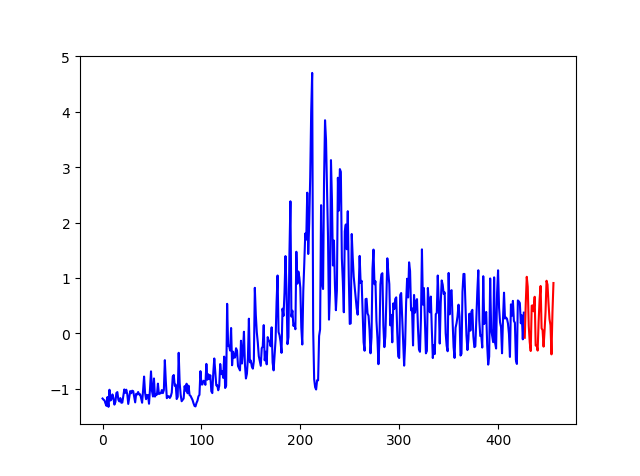bule：true dataset     red：predict为什么选择LSTM（Long short-term memory）？

LSTM网络用来处理带“序列”(sequence)性质的数据。比如时间序列的数据，像每天的股价走势情况，机械振动信号的时域波形，以及类似于自然语言这种本身带有顺序性质的由有序单词组合的数据。
LSTM本身不是一个独立存在的网络结构，只是整个神经网络的一部分，即由LSTM结构取代原始网络中的隐层单元部分。
LSTM网络具有“记忆性”。其原因在于不同“时间点”之间的网络存在连接，而不是单个时间点处的网络存在前馈或者反馈。如下图2中的LSTM单元（隐层单元）所示。图3是不同时刻情况下的网络展开图。图中虚线连接代表时刻，“本身的网络”结构连接用实线表示。LSTM网络最大的特点就是它有输入门、输出门、忘记门三个控制单元（“cell”），随着信息的进入该模型，LSTM中的cell会对该信息进行判断，符合规则的信息会被留下，不符合的信息会被遗忘，这使得它对时间序列的的数据有趋势“记忆”以及预测的能力，大量的训练数据可以使模型“记忆”每年、每月、每周的数据趋势，或者说具有周期性的趋势，这样可以提高时间序列数据预测的准确性。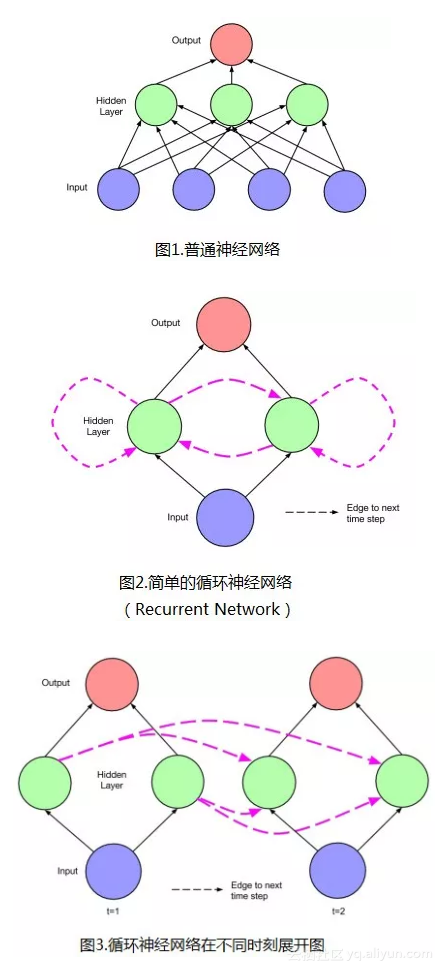如果您对LSTM原理还不太理解请戳：理解LSTM原理

一、数据预处理：

打开dataset你会发现有5个cvs表格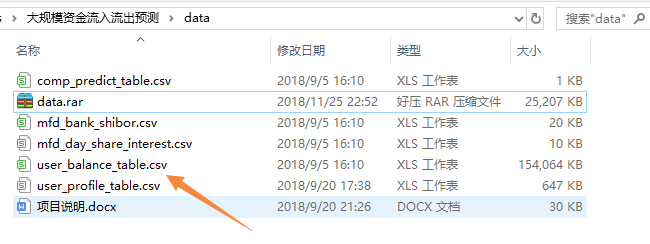但是本例只用到一个表格，就是用户申购赎回数据（user_balance_table.csv）

列名

类型

含义

示例

user_id

bigint

用户 id

1234

report_date

string

日期

20140407

tBalance

bigint

今日余额

109004

yBalance

bigint

昨日余额

97389

total_purchase_amt

bigint

今日总购买量 = 直接购买 + 收益

21876

direct_purchase_amt

bigint

今日直接购买量

21863

purchase_bal_amt

bigint

今日支付宝余额购买量

0

purchase_bank_amt

bigint

今日银行卡购买量

21863

total_redeem_amt

bigint

今日总赎回量 = 消费 + 转出

10261

consume_amt

bigint

今日消费总量

0

transfer_amt

bigint

今日转出总量

10261

tftobal_amt

bigint

今日转出到支付宝余额总量

0

tftocard_amt

bigint

今日转出到银行卡总量

10261

share_amt

bigint

今日收益

13

category1

bigint

今日类目 1 消费总额

0

category2

bigint

今日类目 2 消费总额

0

category3

bigint

今日类目 3 消费总额

0

category4

bigint

今日类目 4 消费总额

0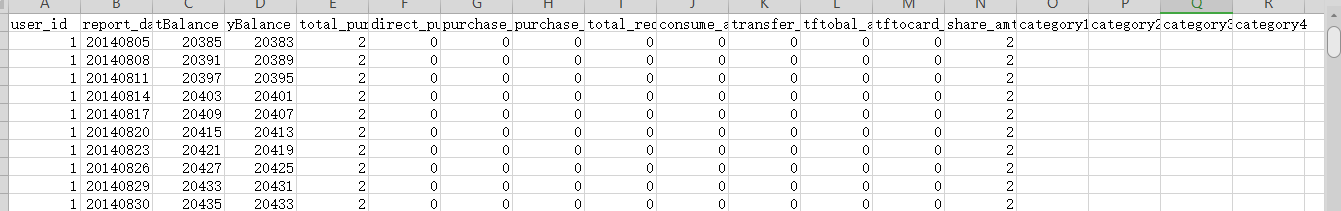1、原始数据集粗处理：先除去14个月总操作数记录低于5次或者总申购值和总赎回值低于10次的用户，

2、剔除无用数据和缺少值处理：检查是否存在缺失值（可以很容易发现这是上表是缺值的）

3、离散化、标准化和均衡化

分别对总申购值、总赎回值按天进行汇总，得到1个2x427维的数据集

在427天中选取一个连续的30天作为测试集（test-data），剩下的作为训练集（train-data）

需要对数据进行预处理，包括：噪音清洗、归一化、编码等

在本例中，作为一个小白，我先试用来，Keras的lstm直接建模，但是在反归一化的时候遇到了关于维度的问题，一时半会没能解决，所以曲线救国我尝试了tensorflow版本的LSTM，算是成了。废话不多说，开始吧！

第一步是将数据以日期整合到一起以便我们可以将日期用作Pandas的索引。

在数据集中还有几个分散的“NA”值；我们现在可以用0值标记它们。

code1： Load_Dataset

# Mingjoy/ 2019/4/20
# -*- coding:utf-8 -*-
import matplotlib.pyplot as plt
import pandas as pd

# Load_dataset
user_balance = pd.read_csv('user_balance_table.csv', parse_dates = ['report_date'])

timeGroup = user_balance.groupby(['report_date']) # groupby()聚类函数,将数据日期整合
purchaseRedeemTotal = timeGroup['total_purchase_amt', 'total_redeem_amt'].sum()  # 累加每一天的申购、赎回总值
purchaseRedeemTotal.plot()
plt.show()

# Save file
purchaseRedeemTotal.to_csv('purchaseRedeemTotal.csv')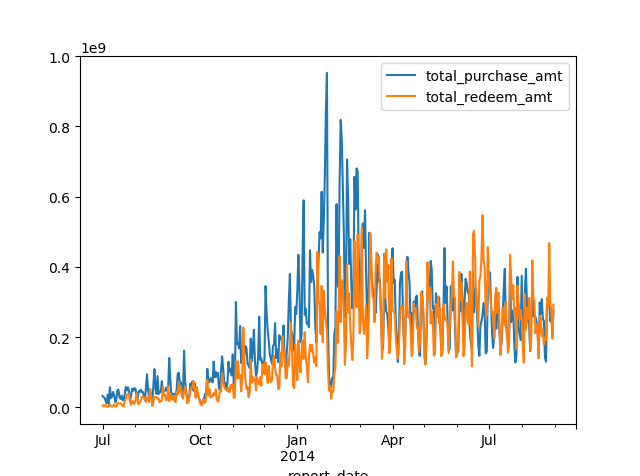为了便于理解LSTM我们先使用一个特征输入，把每日 total_purchase_amt 作为输入特征[x]，后一天的最高价最为标签[y]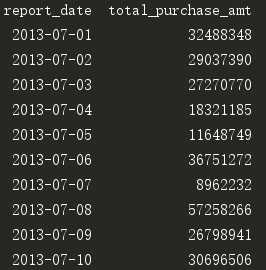#!/usr/bin/env python
# -*- coding:utf-8 -*-

import matplotlib.pyplot as plt
import pandas as pd
import math

# load data(user_balance_table.csv)
user_balance = pd.read_csv('user_balance_table.csv', parse_dates = ['report_date'])
timeGroup = user_balance.groupby(['report_date'])   # groupby()聚类函数
purchaseRedeemTotal = timeGroup['total_purchase_amt', 'total_redeem_amt'].sum()
purchaseRedeemTotal.plot()
plt.show()
# save to file
purchaseRedeemTotal.to_csv('purchaseRedeemTotal.csv')



tensorflow.python.framework.errors_impl.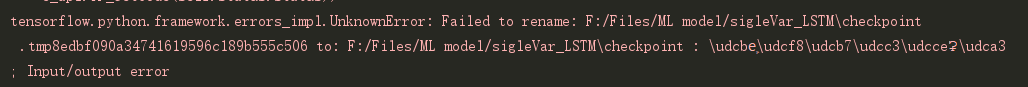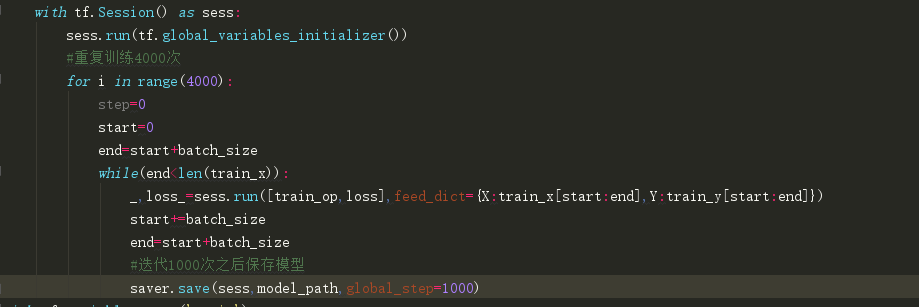代码2

#!/usr/bin/env python
# -*- coding:utf-8 -*-
import numpy
import matplotlib.pyplot as plt
from math import sqrt
from numpy import concatenate
from matplotlib import pyplot
from pandas import read_csv
from pandas import DataFrame
from pandas import concat
from sklearn.preprocessing import LabelEncoder, MinMaxScaler
from sklearn.metrics import mean_squared_error
from keras.models import Sequential
from keras.layers import Dense
from keras.layers import LSTM
import math

# 将序列转换为监督学习问题
def series_to_supervised(data, n_in=1, n_out=1, dropnan=True):
n_vars = 1 if type(data) is list else data.shape
df = DataFrame(data)
cols, names = list(), list()
# input sequence (t-n, ... t-1)
for i in range(n_in, 0, -1):
cols.append(df.shift(i))
names += [('var%d(t-%d)' % (j + 1, i)) for j in range(n_vars)]
# forecast sequence (t, t+1, ... t+n)
for i in range(0, n_out):
cols.append(df.shift(-i))
if i == 0:
names += [('var%d(t)' % (j + 1)) for j in range(n_vars)]
else:
names += [('var%d(t+%d)' % (j + 1, i)) for j in range(n_vars)]
# put it all togethe
agg = concat(cols, axis=1)
agg.columns = names
# drop rows with NaN values
if dropnan:
agg.dropna(inplace=True)
return agg

# 加载数据集
dataset = read_csv('purchaseData.csv', header=0, index_col=0)
dataset = dataset.fillna(value=0)
values = dataset.values
# 整数编码
encoder = LabelEncoder()
values[:, 1] = encoder.fit_transform(values[:, 1])
# 确保所有数据是浮动的
values = values.astype('float32')
# 归一化特征
scaler = MinMaxScaler(feature_range=(0, 1))
scaled = scaler.fit_transform(values)

# 构建监督学习问题
reframed = series_to_supervised(scaled, 5, 5)
print(reframed.shape)                        # output:（418，120）

# 分为训练集和测试集
values = reframed.values
n_train_days = 397
train = values[:n_train_days, :] # day1 to day397
test = values[n_train_days:, :]  # day398 to day 427
# 分为输入和输出
train_X, train_y = train[:, :-1], train[:, -1]
test_X, test_y = test[:, :-1], test[:, -1]
print(train_X.shape, len(train_X), train_y.shape)     # (397, 119) 397 (397,)

# 重塑为3D形状 [samples, timesteps, features]
train_X = train_X.reshape((train_X.shape, 1, train_X.shape))
test_X = test_X.reshape((test_X.shape, 1, test_X.shape))
print(train_X.shape, train_y.shape, test_X.shape, test_y.shape) #(397, 1, 119) (397,) (21, 1, 119) (21,)

# 设计网络
model = Sequential()
model.add(LSTM(50, input_shape=(train_X.shape, train_X.shape)))
model.add(Dense(1))
model.compile(loss='mae', optimizer='adam')
# 拟合神经网络模型
history = model.fit(train_X, train_y, epochs=50, batch_size=72, validation_data=(test_X, test_y), verbose=2,
shuffle=False)
# 绘制历史数据
pyplot.plot(history.history['loss'], label='train')
pyplot.plot(history.history['val_loss'], label='test')
pyplot.legend()
pyplot.show()

print(test_X.shape) #(21, 1, 119)
# 作出预测
yhat = model.predict(test_X)
test_X = test_X.reshape((test_X.shape, test_X.shape))
print(yhat.shape)   #(21, 1)
print(test_X.shape) #(21, 119)

# 反向缩放预测值
inv_yhat = concatenate((yhat, test_X[:, 1:]), axis=1)
print(inv_yhat.shape)#(21, 119)
# inv_yhat = inv_yhat.reshape((len(inv_yhat),12))
inv_yhat = inv_yhat.reshape((test_X.shape, test_X.shape))
inv_yhat = scaler.inverse_transform(inv_yhat)  # X : array-like, shape [n_samples, n_features]
inv_yhat = inv_yhat[:,0]
# 反向缩放实际值
test_y = test_y.reshape((len(test_y), 1))
inv_y = concatenate((test_y, test_X[:, 1:]), axis=1)
inv_y = scaler.inverse_transform(inv_y)       # X : array-like, shape [n_samples, n_features]
inv_y = inv_y[:,0]

# 计算RMSE
rmse = sqrt(mean_squared_error(inv_y, inv_yhat))
print('Test RMSE: %.3f' % rmse)
plt.plot(inv_yhat)
plt.plot(yhat)
plt.show()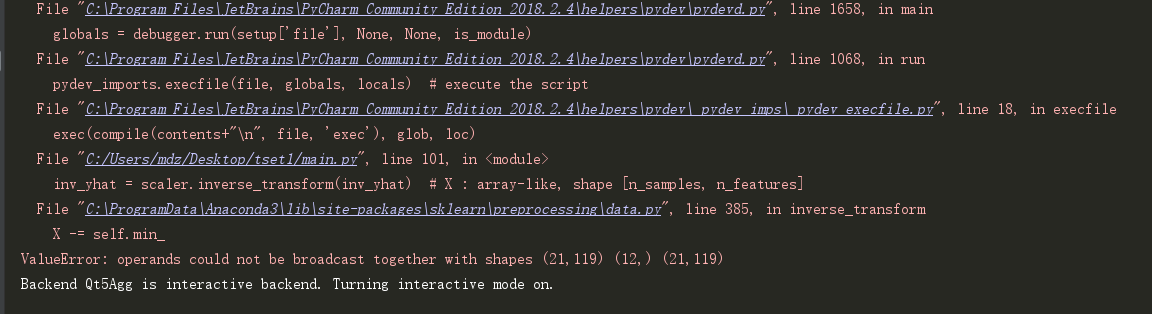代码3

import pandas as pd
import numpy as np
import matplotlib.pyplot as plt
import tensorflow as tf
import csv
import codecs

#——————————————————导入数据——————————————————————
f=open('purchase.csv')
df=pd.read_csv(f)
data=np.array(df['total_purchase_amt'])   #获取最高价序列
#以折线图展示data
plt.figure()
plt.plot(data)
plt.show()
normalize_data=(data-np.mean(data))/np.std(data)  #标准化
normalize_data=normalize_data[:,np.newaxis]       #增加维度

#生成训练集
#设置常量
time_step=20      #时间步
rnn_unit=10       #hidden layer units
batch_size=60     #每一批次训练多少个样例
input_size=1      #输入层维度
output_size=1     #输出层维度
lr=0.0006         #学习率
train_x,train_y=[],[]   #训练集
for i in range(len(normalize_data)-time_step-1):
x=normalize_data[i:i+time_step]
y=normalize_data[i+1:i+time_step+1]  #后一天的total_purchase_amt最为标签[y]
train_x.append(x.tolist())
train_y.append(y.tolist())

def data_write_csv(file_name, datas):#file_name为写入CSV文件的路径，datas为要写入数据列表
file_csv = codecs.open(file_name,'w+','utf-8')#追加
writer = csv.writer(file_csv, delimiter=' ', quotechar=' ', quoting=csv.QUOTE_MINIMAL)
for data in datas:
writer.writerow(data)
print("保存文件成功，处理结束")

#——————————————————定义神经网络变量——————————————————
X=tf.placeholder(tf.float32, [None,time_step,input_size])    #每批次输入网络的tensor
Y=tf.placeholder(tf.float32, [None,time_step,output_size])   #每批次tensor对应的标签
#输入层、输出层权重、偏置
weights={
'in':tf.Variable(tf.random_normal([input_size,rnn_unit])),
'out':tf.Variable(tf.random_normal([rnn_unit,1]))
}
biases={
'in':tf.Variable(tf.constant(0.1,shape=[rnn_unit,])),
'out':tf.Variable(tf.constant(0.1,shape=[1,]))
}

#——————————————————定义神经网络变量——————————————————
def lstm(batch):      #参数：输入网络批次数目
w_in=weights['in']
b_in=biases['in']
input=tf.reshape(X,[-1,input_size])  #需要将tensor转成2维进行计算，计算后的结果作为隐藏层的输入
input_rnn=tf.matmul(input,w_in)+b_in
input_rnn=tf.reshape(input_rnn,[-1,time_step,rnn_unit])  #将tensor转成3维，作为lstm cell的输入
cell=tf.nn.rnn_cell.BasicLSTMCell(rnn_unit)
init_state=cell.zero_state(batch,dtype=tf.float32)
output_rnn,final_states=tf.nn.dynamic_rnn(cell, input_rnn,initial_state=init_state, dtype=tf.float32)  #output_rnn是记录lstm每个输出节点的结果，final_states是最后一个cell的结果
output=tf.reshape(output_rnn,[-1,rnn_unit]) #作为输出层的输入
w_out=weights['out']
b_out=biases['out']
pred=tf.matmul(output,w_out)+b_out
return pred,final_states

#——————————————————训练模型——————————————————
def train_lstm():
global batch_size
pred,_=lstm(batch_size)
#损失函数
loss=tf.reduce_mean(tf.square(tf.reshape(pred,[-1])-tf.reshape(Y, [-1])))
train_op=tf.train.AdamOptimizer(lr).minimize(loss)
saver=tf.train.Saver(tf.global_variables())   # 创建一个Saver对象
# base_path = r'C:\Users\mdz\Desktop\Forecast\lstm'
with tf.Session() as sess:
sess.run(tf.global_variables_initializer())
#重复训练10000次
for i in range(4000):
step=0
start=0
end=start+batch_size
while(end<len(train_x)):
_,loss_=sess.run([train_op,loss],feed_dict={X:train_x[start:end],Y:train_y[start:end]})
start+=batch_size
end=start+batch_size
#迭代1000次之后保存模型
saver.save(sess,'C:\\Users\\mdz\\Desktop\\Forecast\\my_test_model',global_step=1000)

with tf.variable_scope('train'):
train_lstm()
print('train finsh')
#————————————————预测模型————————————————————
def prediction():
pred,_=lstm(1)      #预测时只输入[1,time_step,input_size]的测试数据
saver = tf.train.Saver(tf.global_variables())  # 创建一个Saver对象
"""
导入一个预先训练模式：
a）创建网络结构图： 导入原始网络结构图
b）加载的（变量）参数： 使用restore()方法恢复模型的变量参数。
"""
with tf.Session() as sess:
# saver = tf.train.import_meta_graph(r'C:\Users\mdz\Desktop\Forecast\my_test_model-1000.meta')
saver .restore(sess,tf.train.latest_checkpoint('./'))

#取训练集最后一行为测试样本。shape=[1,time_step,input_size]
prev_seq=train_x[-1]
predict=[]
#得到之后30个预测结果
for i in range(30):
next_seq=sess.run(pred,feed_dict={X:[prev_seq]})
predict.append(next_seq[-1])
#每次得到最后一个时间步的预测结果，与之前的数据加在一起，形成新的测试样本
prev_seq=np.vstack((prev_seq[1:],next_seq[-1]))
#以折线图表示结果
plt.figure()
plt.plot(list(range(len(normalize_data))), normalize_data, color='b')
plt.plot(list(range(len(normalize_data), len(normalize_data) + len(predict))), predict, color='r')
plt.show()
# 将预测数据保存到文件中
print(predict)

with tf.variable_scope('train',reuse=True):
prediction()


Reference

《TensorFlow: 实战Google深度学习框架》 对于小白规范化自己的代码，增强代码的复用性很有必要看看

使用Keras进行LSTM实战

Tensorflow实例：利用LSTM预测股票每日最高价（一）

Tensorflow实例：利用LSTM预测股票每日最高价（二）


展开全文• 　一直想写一篇面对初学者的文章从上层应用到底层的机器学习基础方面全流程的阐释当下流行的深度学习算法（CNN，RNN等）。刚好春节前有同学工作内容涉及对话机器人，我也从零开始接触学习了下，所以就借这个案例展开...
• 　前几篇文章中已经介绍了我们的seq2seq模型使用均方误差作为损失函数，使用SGD算法（随机梯度下降）算法来训练模型参数，实例中代码如下： net = tflearn.regression(real_output_sequence, optimizer='sgd', ...
• 理论与实战结合， 基于深度学习主流框架Tensorflow实例演示如何用深度学习来进行文本分类任务，其中涉及深度学习主流架构LSTM模型以及自然语言处理中流行的word2vec词向量建模方法，分模块解读如何用框架一步步完成...自然语言处理 人工智能 深度学习
• 提出基于长短时记忆(LSTM)神经网络构建的突水预测模型，将煤矿突水实例的数据作为样本数据对模型进行训练。最后，将LSTM神经网络模型与遗传算法–反向传播(GA-BP)神经网络模型和反向传播(BP)神经网络模型进行对比...
• 理论与实战结合， 基于深度学习主流框架Tensorflow实例演示如何用深度学习来进行文本分类任务，其中涉及深度学习主流架构LSTM模型以及自然语言处理中流行的word2vec词向量建模方法，分模块解读如何用框架一步步完成...自然语言处理 深度学习 人工智能
• 理论与实战结合， 基于深度学习主流框架Tensorflow实例演示如何用深度学习来进行文本分类任务，其中涉及深度学习主流架构LSTM模型以及自然语言处理中流行的word2vec词向量建模方法，分模块解读如何用框架一步步完成...自然语言处理 深度学习 人工智能
• 理论与实战结合， 基于深度学习主流框架Tensorflow实例演示如何用深度学习来进行文本分类任务，其中涉及深度学习主流架构LSTM模型以及自然语言处理中流行的word2vec词向量建模方法，分模块解读如何用框架一步步完成...自然语言处理 深度学习 人工智能
• 理论与实战结合， 基于深度学习主流框架Tensorflow实例演示如何用深度学习来进行文本分类任务，其中涉及深度学习主流架构LSTM模型以及自然语言处理中流行的word2vec词向量建模方法，分模块解读如何用框架一步步完成...自然语言处理 深度学习 人工智能
• 它们可以用于以快速的方式创建新实例，而手动创建新实例的成本更高，或者也不可能。它们可以模拟一些未被记录或遗漏的情况。它们可以用来生成不同的副本，这些副本与原始副本不同，具有一定程度的噪音，从而拥有足够...人工智能 时间序列生成
• 理论与实战结合， 基于深度学习主流框架Tensorflow实例演示如何用深度学习来进行文本分类任务，其中涉及深度学习主流架构LSTM模型以及自然语言处理中流行的word2vec词向量建模方法，分模块解读如何用框架一步步完成...自然语言处理 深度学习 人工智能
• ## CART回归树及其实例

千次阅读 2019-05-11 11:01:13
当前，最火的两类算法莫过于神经网络算法（CNN、RNN、LSTM等）与树形算法（随机森林、GBDT、XGBoost等），树形算法的基础就是决策树。决策树因其易理解、易构建、速度快的特性，被广泛应用于统计学、数据挖掘、机器...
• 一、用词嵌入，rnn、lstm算法，可以在很小的训练集的基础上，建立精确的分类器 1、简单的baseline 构建数据集： 小的数据集（X,Y）其中，X包含127个句子(字符串)，Y包含0-4之间的标签，代表表情符号 训练数据集...
• 最近开始啃LSTM，发现BPTT这块还是不是很清晰，结合RNN，把这块整理整理 RNN前馈神经网络（feedforward neural networks）如下图所示（这块内容可见我的博客神经网络BP算法）： 假设我们的训练集只有一个实例（x...神经网络 深度学习
• 本文将LSTM用于短期电力负荷预测 , 提出基于LSTM的短期电力负荷预测模型 , 同时建立布谷鸟算法模型对 LSTM进行参数优化以提高预测精度, 并以浙江某地区的历史负荷数据和气象数据为例进行验证 , 实例验证表明 , CS-...机器学习 时间序列预测 变分模态分解 最小二乘支持向量机
•  本篇文章是用来做大作业的，会讲到神经网络基础、逆反馈算法推导、循环神经网络和LSTM基础（这里关于LSTM是参考【1】）以及它们的用于股市预测的实例。神经网络（NeuralNetworks）是一种用训练数据拟合目标函数的...
• 长短时记忆网络LSTM在针对短时时间序列预测问题上近来年受到大家的关注，但由于该方法为深度...为此，本文将采用改进的麻雀搜索算法ISSA对 LSTM进行参数优化 , 并用实例验证表明 , ISSA-LSTM 模型的预测效果。 ...机器学习 深度学习 matlab
• 对此,基于深度信念网络(DBN)和支持向量回归机(SVM),提出城轨交通短时客流深层预测模型(DBN-P/GSVM),同时基于遗传算法(GA)和粒子群算法(PSO)实现SVM的参数寻优.最后,对成都地铁火车北站客流量预测进行实例分析.结果...
• 解析模式 该模块从文本和实体列表中提取模式。 目前，我们不会尝试检测文本中的坐标，但是可以存储句子索引。... 如果网络是由人（或后来的机器学习算法，如LSTM + RN）解释的，则这不是问题 去做： 维护 事故JavaScript
• LSTM，seq2seq，再到注意力机制，层层递进详解各个模型的理论基础和代码实例。 7. 深度学习实践技巧 这部分介绍深度学习的实战步骤中的一些关键问题。 8. 自然语言处理 关于自然语言处理的课程笔记或者实例。 9. ...
• 为了解决此问题，我们提出了一种基于长短期记忆（基于LSTM）的实时流量预测算法TrafficPredict。 我们的方法使用实例层来学习实例的移动和交互，并使用类别层来学习属于同一类型的实例的相似性以完善预测。 为了...
• 本书从深度学习原理入手，由浅入深，阐述深度学习中神经网络、深度神经网络、卷积神经网络、自编码器、循环神经网络等，同时穿插学习PyTorch框架的各个知识点和基于知识点的实例。最后，综合运用PyTorch和深度学习...